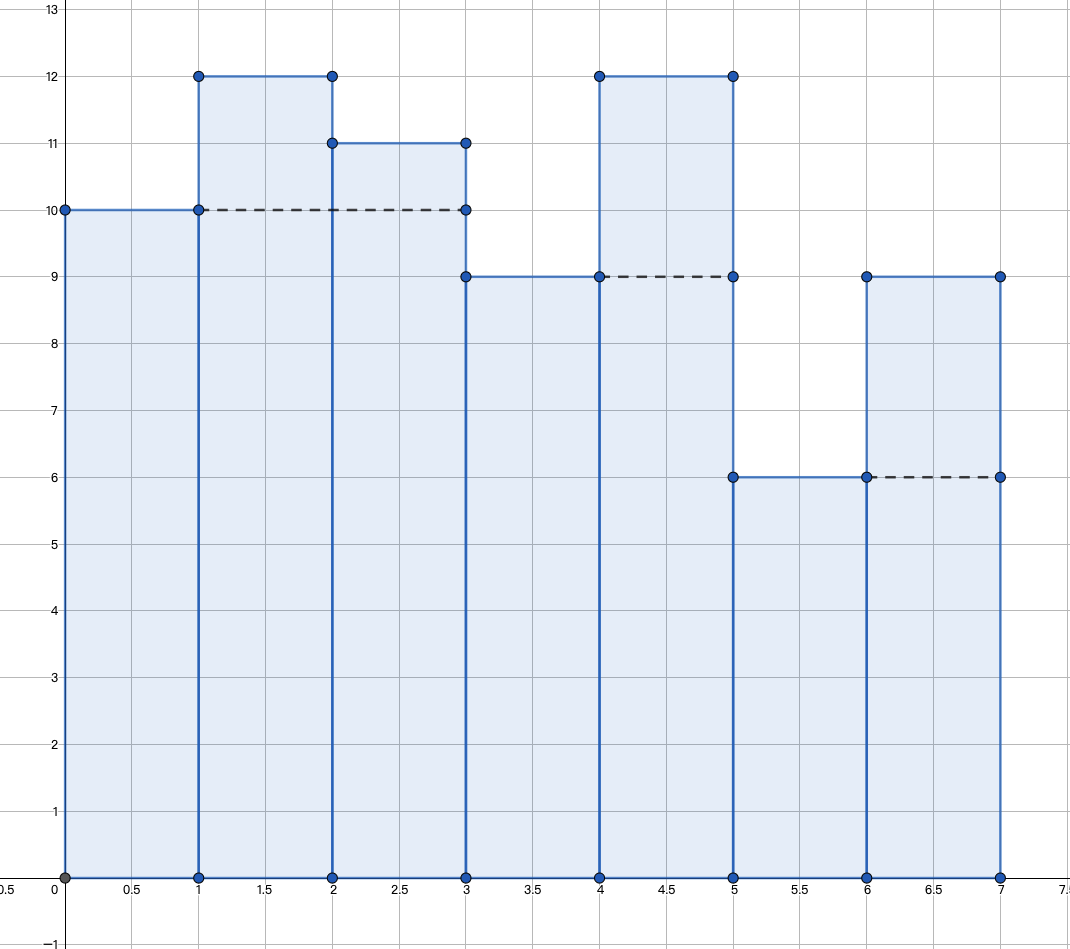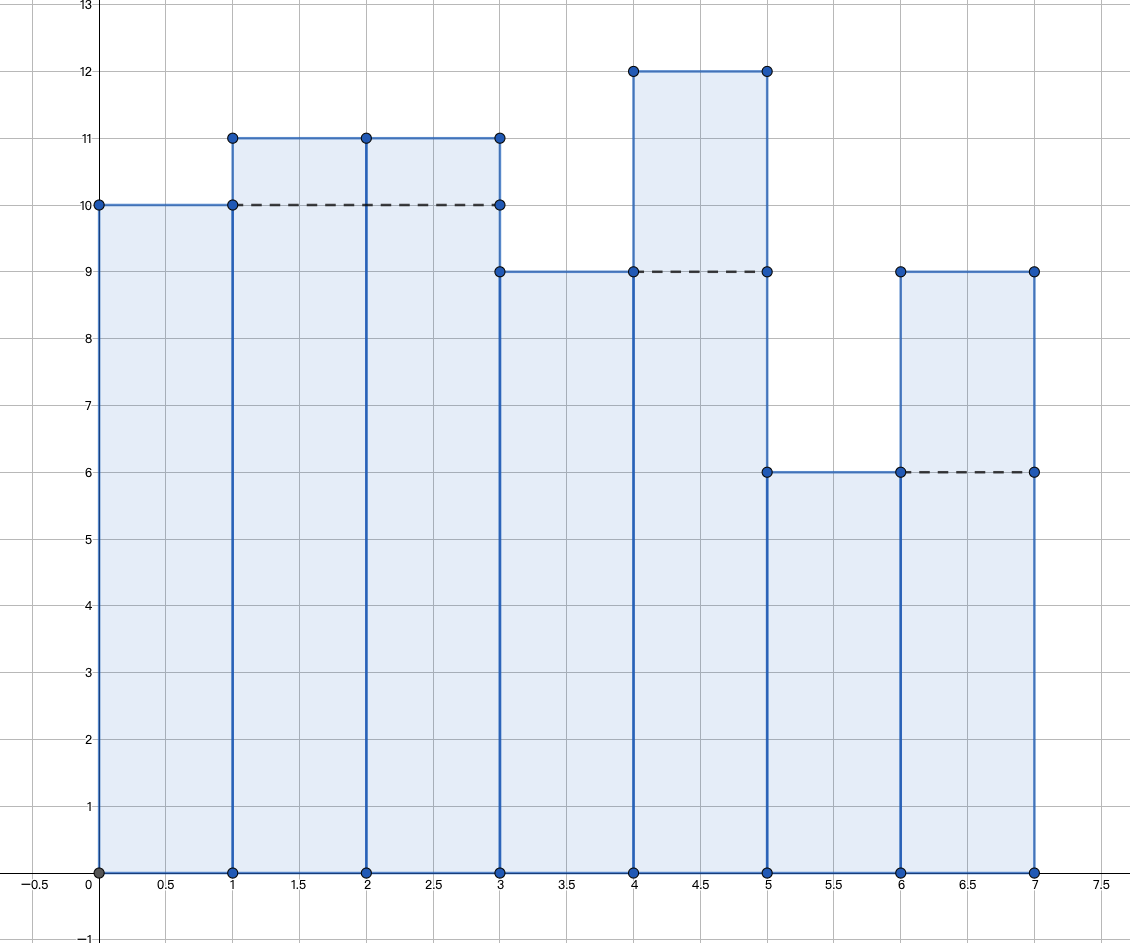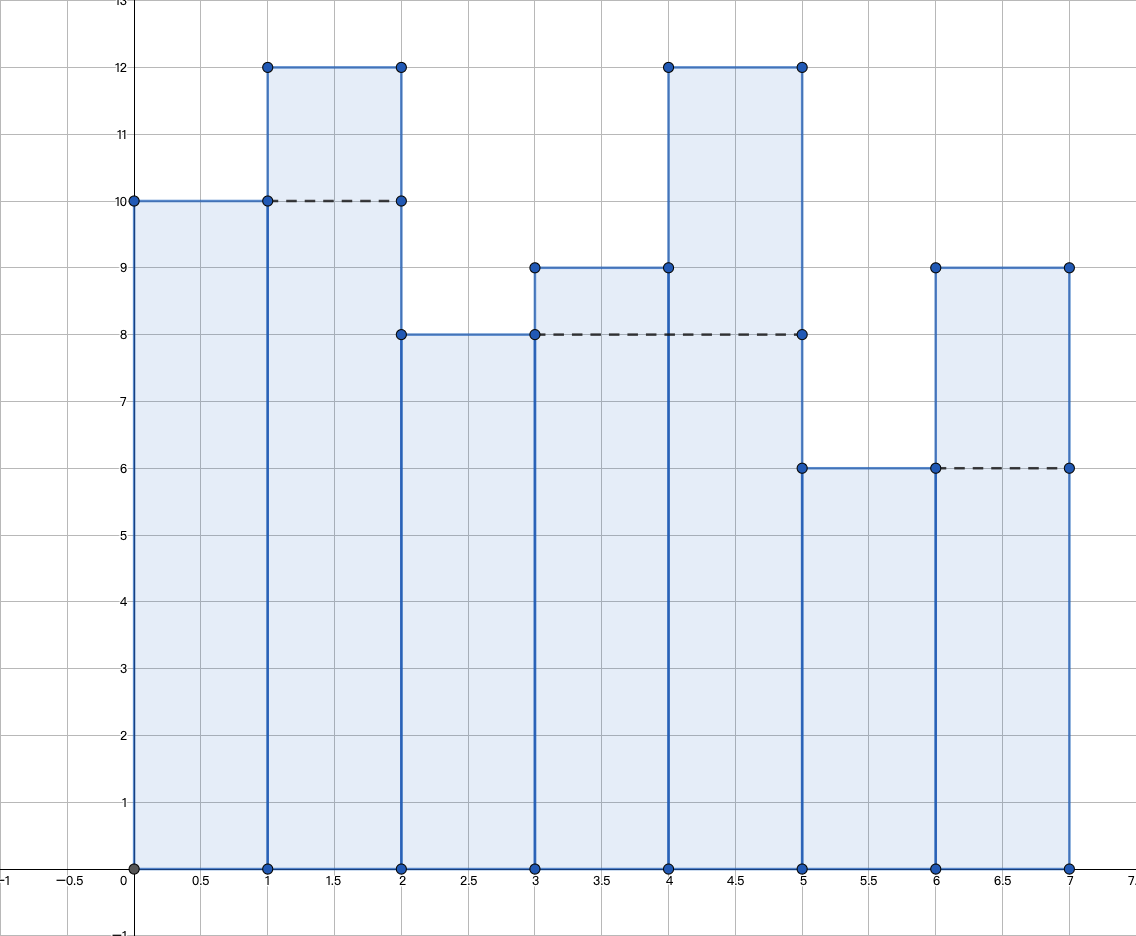# B. Array Decrements

## 题目解析

• 如果 $b_i \neq 0$ ，那么 $delta = a_i - b_i$
• 如果 $b_i = 0$ ，那么 $delta \geq a_i - b_i$

# C. Restoring the Duration of Tasks

## 题目解析

• 如果 $i = 1$，那么 $task_i$ 的实际开始时间是 $task_i$ 的到达时间，即 $s_i$
• 如果 $i \neq 1$ ，那么 $task_i$ 的实际开始时间是 $task_{i - 1}$ 的结束时间和 $task_i$ 的开始时间的最大值，即 $\max(s_i, f_{i - 1})$

# E. Price Maximization

## 题目解析

$\lfloor\frac{a_i + a_j}{k}\rfloor = \lfloor\frac{a_i}{k}\rfloor + \lfloor\frac{a_j}{k}\rfloor + \lfloor\frac{a_i \bmod k + a_j \bmod k}{k}\rfloor$

# G. Count the Trains

## 题目解析PS: 学过一点珂学，很容易就能想到。

## 参考代码

Codeforces Round 797 (Div. 3)
https://dianhsu.com/2022/06/08/cf1690/

Dian Hsu

2022年6月8日